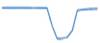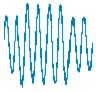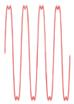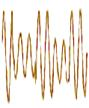# TECHNICAL TIPS

Whether you have a question or just want to browse, take a few moments to read over our collection of commonly asked technical questions and help tips. You might find what you’re looking for without even contacting our support staff. These can help you get started and provide on-going reference.

### PU (Per Unit) Explained

PU stands for Per Unit. It is a term that electric utility engineers frequently use, and it was incorporated into IEEE 1159.
PU is the percentage formed by dividing the actual voltage by the nominal. For example, a sag to 0.8 pu on a 480V circuit would be a sag to 366V, and an interruption to 0.1 pu on a 120V circuit would be 12V.
The idea of “per unit” is to express a voltage without regard to the nominal, since through the various transformers in an electric utility system, the percent change will be similar (less losses in the transformer). If the 138kv transmission line sags to 0.7 pu, then the 13kv distribution line will see a similar sag, as will the end user voltage. (Of course, if there are delta-wye or wye-delta transformers in between, this will change the values, but the concept remains the same.)

### Electrical Harmonic Power Flow Direction

Most people in the power engineering field have encountered the question about which direction the electrical harmonic power is flowing, from the source to the load, or, from the load to the source. While this is still a controversial topic for some people, the most commonly accepted practice for determining this is to look at the harmonic watts phase angle, or the relationship between the voltage and current for a particular harmonic. The same rules that would be applied to a pure sine wave of voltage and current (which has only a fundamental frequency component) would be applied here.
The phase relationship between voltage and current with a pure resistive load is zero degrees, or a power factor of 1. If the load is a pure inductor, then the current lags the voltage by 90 degrees, which is normally displayed as a +90 degrees. If the load is pure capacitor, the current leads the voltage by 90 degrees, so the phase angle is called -90 degrees. This is so that the power factor of an inductive and resistive load would be a positive number between 0 and 1, while a capacitive and resistive load would be a negative number.
If the phase angle between the voltage and current is more than 90 degrees apart, this usually means that the current probe used with a power/harmonic meter or analyzer is placed in opposite direction of the assumed power flow. Most current probes have an arrow that should be pointing in the direction from the source to the load, which is the normal direction of power flow. When the phase angle of the harmonic voltage and current is between 90 degrees and 270 degrees (270 is also referred to as -90 degrees) on a properly installed CT, then it is assumed that this harmonic power flow is in the opposite direction of the fundamental power flow, or from load to source.
In some Dranetz products, this is indicated by the words SOURCE or LOAD next to the printout of each harmonic watt. In other products, you have to look at the phase angle of the harmonic watts to determine where it falls. The user must be cautioned that in a number of measurements, the harmonic current and voltage levels are so low that the harmonic watts number is so small that it may be meaningless, as would be the direction of power flow information. For example, if on a 120V/30A circuit, there is a 5th harmonic voltage of 0.05 V and harmonic current of 0.2 A, 0.01W is really insignificant and the accuracy of the direction is very low.

### Source Stiffness

“Stiffness” of the source is kind of an engineering slang term for the ability of the source to provide a nearly constant voltage level even under heavy current loading conditions. In technical terms, it is related to the equivalent source impedance. Ohm’s and Kirchoff’s Laws are the keys here.

For example, if the source has a 1 ohm impedance and is supplying 100V, then if the load draws 1A, then the load will see 99V, with 1V dropped across the source impedance. If the load draws 10A, then the load will see only 90V, with 10V dropped across the source impedance. However, if the source impedance were 0.1 ohms, 10A draw by the load would still give the load 99V, as only 1V would be dr
opped across the source impedance. Hence, the source with the 0.1ohm source impedance is a much stiffer source than the 1ohm source. This is true of harmonic source impedances as well. Typically, the stiffer the source, the less likely is it that the user will have power quality problems, whether they be harmonic, RMS variations like sags, etc. Though like any rule, there are exceptions.

### Harmonic Magnitudes

Harmonics are often displayed as a harmonic spectrum, which is either a list or bar graph showing the magnitude of each harmonic, for voltage and current. The magnitudes are a good clue as the source of the harmonics. If the current harmonics have a significant 3rd harmonic, slightly smaller 5th, even smaller 7th and so on, this is often caused by single phase, rectified-input, switching power supplies, such as in computers, printers, and other information technology equipment found in office environments. If the dominant harmonics are the 5th and 7th, then the 11th and 13th, then the 17th and 19th, then the source is often a 6 pulse or pole converter, also known as a 3 phase, full wave rectifier, which is found in adjustable speed drivers and other larger electronic loads.

### Harmonic Pollutions

It is usually the harmonic currents that are of concern, as they can cause harmonic pollution to spread to other equipment. Just like Current * Impedance = Voltage for fundamental frequency, Ohms Law also applies to harmonic current, impedance and voltage. Loads that draw current in a non-linear manner will cause harmonic-rich current to react with harmonic impedances and generate harmonic voltages that other loads will see. Harmonic impedances can change values with frequency or the harmonic number, often increasing significantly with the higher harmonics. This means that it will take less harmonic current to produce a significant harmonic voltage.

### The sum of THD

A common statistical number used is called THD, or total harmonic distortion. This is a mathematical process where: the magnitude of the harmonics for voltage or current are squared; summed; the square root is taken on the sum; and the result is the divided by either the fundamental RMS or the total RMS value; and lastly, multiplied by 100%. This number can different significantly based on the divisor. (Typically, Fundamental is used in North America, and Total in Europe) Using THD for current can be very misleading and should generally be avoided. The actual magnitudes of the harmonic current are more meaningful. If there is very little magnitude of harmonic current, as in the neutral of a wye circuit, then the THD can be very large and it still wouldnt be a problem. For example, 0.5A of current in the neutral of a 30A circuit could be made of 0.25 A fundamental, and 0.25 A of 3rd harmonic. This would yield a 100% THD, which sounds bad, but is really insignificant on a 30A circuit./su_spoiler]

### Common Harmonics

Harmonics are typically defined as frequencies that are integer multiples of the fundamental frequency. For 60Hz power systems, these means that the 2nd harmonic is 120Hz, the third harmonic is 180Hz, the fourth is 240 Hz, , the nth harmonic is n*60. Frequencies are found that are actually in between these harmonic frequencies are called interharmonics (such as 185Hz), but are generally much less common than harmonic frequencies themselves. Frequencies below the fundamental frequencies are called subharmonics (such as 9Hz), and often contribute to the phenomena of light flicker.

### RMS Sags

Variations in the RMS value are often used to trigger capturing of PQ data. The most common type of RMS variation is the sag, (or dip in European lingo). Some studies show over 60% of the PQ disturbances are sags, which is when the RMS value goes below 90% of the nominal value. On a typical office or residential outlet, that would be dropping from 120Vrms to 108Vrms. If the voltage goes down below 10% of nominal, we call that an interruption. Conversely, if it increases above 110% of nominal, that is a swell.

### Electromagnetic Phenomena

The most common type of electromagnetic phenomena that cause power quality related problems are changes in the basic waveshape of the voltage  the sine wave. One mathematical number used to represent this complex shape with a single number is RMS  root mean squared. It takes each of the sample points (typically 128) in one cycle of the waveform, multiplies the value by itself (squares it), adds them all up and takes the average (mean), and then takes the square root of that number. This is different than the peak value, which is the largest sample value in a cycle. For different waveshapes, there are different relationships between the peak and RMS. For sine waves, the peak is 1.414 times larger than the RMS value, or the RMS value is 0.707 times smaller than the peak. This relationship doesnt hold with distorted waveforms, such as when harmonics are present, which is why you should use a true RMS meter, not one that multiplies the peak value times 0.707 to determine RMS.

### Loose Connections

If you have taken a distribution panel off for whatever reason, it is a good time to use one of the most important power quality tools, the screwdriver. Be sure to wear the proper safety equipment and to follow all necessary safety precautions. In most facilities, the current flows during only part of the day. Today, this current is often contains heat-generating harmonic currents. The heating/cooling/heating/cooling cycle and resulting expansion and contraction of the wires can cause the connections to loosen over time. This loosening increases the impedance of the connection, which further increases the heating effects. Tightening loose connections with the screwdriver can help reduce voltage drops and minimize the fire potential.

### Harmonic Differences

Harmonics are typically grouped by their harmonic number, either odd or even. Odd are the 3, 5, 7, 9, etc and even being the 2,4,6,8,and so on. There is another grouping used, called the triplens, which are the 3, 6, 9, 12, etc. The triplens are so grouped because triplen harmonic currents will add in the neutral of a three-phase, four wire wye circuit, as opposed to canceling out. This has resulted in the need to make the neutral conductor equal to or up to 1.73 times as large in current-carrying-capability as the phase conductors. Even harmonics are grouped together as they typically arent found in systems with properly functioning equipment, unless there are half-wave rectifiers present as loads. If half of the input rectifiers arent functioning properly in a full wave rectifier, then the load will draw current as if it is a half wave rectifier, and the current waveform will be rich in even harmonics. This is a clue that something maybe broken. Even harmonics are recognizable in a waveform as they cause a loss in symmetry within the halves of the waveform.

### Power Factors

Power factor is another parameter that is affected by power quality phenomena, particularly distortion and imbalance. This creates even more confusion about the term true power factor. Power factor is a measure of how efficiently a load uses the electricity, or, how much energy is consumed by the load versus how much the electricity provider must deliver. This has been defined as real power divided by apparent power, watts / volt-amperes, or W / VA. Until the onslaught of rectified input type loads (also called switching power supplies, or electronic or non-linear loads), most electrical loads were resistive and/or inductive loads, such as heaters, incandescent lights, and electrical motors. Whereas the voltage and current may not have been exactly in phase, both were nearly sinusoidal in their waveshapes, having only fundamental frequency components present. Hence, real power being equal to Vrms * Irms * cos (angle between V & I called theta) and apparent power being Vrms * I rms reduced down to Power Factor equal to Cos (angle theta). People then assumed that this was the real formula for PF, and revenue meters used such for billing purposes.
As the rectified input loads began to become the norm, the current waveshape in particular lost its sinusoidal shape, becoming rich in other harmonic frequency components. SCR-gated loads conducted current only during part of the voltage waveform. Even if the fundamental frequency components were in-phase, the real power was no longer just the Vrms * I rms * cos (theta), since each of the harmonic voltages and currents could have a different value for theta. Not surprising, watts become a lower value, since the purpose of the rectified input switching power supplies and SCR-gated loads was to reduce the real power being consumed. But the apparent power, Vrms * Irms, was still the same. So, surprising as it may be to some people, the power factor become smaller. In one example, a utility person replaced an old electromechanical meter with a new one that called PF with the traditional W/VA method and now the customer owed for a PF penalty (which the customer refused to pay since they hadnt changed their loads).

### High Even Harmonics

The presence of a high percentage of even harmonics usually indicates that there is a significantly large half-wave rectifier on the circuit, or that a full-wave rectifier is damaged and is acting like a half-wave rectifier.
Odd harmonics are usually make up the majority of the spectrum on most electrical circuits. Even harmonics in significant proportions are usually not found, except where current is being drawn on only half the cycle. The Fourier expansion of a half-wave rectified signal is composed entirely of even harmonics, whereas the typical electronic loads, such as PCs, laser printers, or ASDs, have predominately odd harmonic spectrums. Even harmonics are often detectable by the lack of quarter-wave symmetry of the waveform. This means that the part of the waveform up to the first peak of the sine wave doesnt look like the mirror image of the 2nd part of the waveform, as it goes from the peak back to the zero axis. The same dis-symmetry will show up between the 3rd and the 4th parts in the negative half cycle of the waveform.

### Causes of Transients

Transients are very short duration disturbances, less than 1/4 cycle of power frequency and more often, measured in microseconds. They used to be referred to as impulses, surges, spikes or glitches. But those terms can have ambiguous meanings, so the term transient was adopted by the IEEE and other standards groups. Common causes of voltage transients are power factor capacitor banks being switched on or off, lightning striking a conductor or adjacent to a conductor, arcing from a phase conductor coming in contact with some sort of ground potential (such as a tree), and the notches resulting from the commutation period of the SCRs on rectified input 3 phase power supplies (such as in ASDs). Possible effects of transients include data corruption on memory devices, equipment damage, data transmission errors, intermittent equipment operation, reduced equipment life, and irreproducible problems. Transients are often sneaky, in that they happen very quickly and randomly, and many power quality monitors will not capture them, especially the higher frequency transients.

### Causes of Swells

Swells are increases in the voltage, typically above 110% of the nominal. Though must less common than sags, swells can cause catastrophic failures in equipment if the voltage exceeds the safe input level of the equipment for too long. Swells can be caused when a large load is suddenly turned off (opposite of the cause of sags). The voltage will increase for 30-60 cycles, until the automatic tap changers can bring the voltage back into normal regulation limits.

### Causes of Sags

Sags are often caused by sudden, large increases in current, which causes a proportional voltage drop in the wiring, leaving less voltage remaining for the loads. If it is a fault on the electric distribution system, such as a phase-to-ground short circuit caused by lightning, animals, tree branches, or accidents, then the direction of the sag is called upstream or source side, or towards the generating source. If a load starts up, such as a large HP motor, then the direction of the sag is said to be downstream or load side. If the remaining voltage during the sag is too low for the equipment to operate properly, the process can be interrupted or corrupted. Though equipment is usually not damaged during such, the product being produced often has to be scrapped, and there may be a significant restart time to get the operation running smooth again.

### Flickering Lights

Some changes or modulations of the voltage that arent large enough to be consider sags may not seem to effect equipment operation. However, these anomalies can result in quality variations in extrusion and textile processes and in flickering lights that can cause human discomfort. In the case of flicker, the frequency of the modulation is critical as to whether it will be noticeable to the particular susceptibilities of the human eye and brain. For example, it would only take about 0.3 volts of modulation at 9Hz on a 120V system for most people to notice the flicker in a 60W light bulb. However, at 1 Hz, it would take nearly 10 times the modulation to be noticeable.

# Power Quality Disturbance Definitions

Power Quality Disturbance Definitions, Classifications and Applicable Standards
All disturbances relate to voltage unless otherwise stated.

 Incident Type Definition Graphic representation Applicable standards Magnitude (regulation) Long term level of voltage established through transformer taps and dynamically controlled by regulators. Measurement of voltage magnitude over the long term serves the basis for the capture and characterization of other power quality disturbances.Presently specified in TSC Appendix 2:and the IESO Market Rules Appendix 4.1:Voltages below 50kV are maintained in accordance with CAN/CSAC235. Essentially, systems below 50kV, voltage levels must be regulated to within +/-6% of nominal voltage. Unbalance Condition of the 3 phase power system where the RMS magnitude or phase angle of the line voltages are not equal. This is usually determined as a percent of the ratio of negative sequence component to positive sequence component.Presently specified in TSC Appendix 2, Transmission system unbalances are limited to 2% (CAN/CSA E 1000 2-2-97). Harmonics Components of electricity with frequencies which are an integral multiple of the fundamental frequency (60Hz). This is usually expressed in Total Harmonic Distortion or as individual harmonic order (frequency) components.Presently specified in TSC Appendix 2as respecting the limitations in Table 11.1 Voltage Distortion Limits Std. IEEE 519-1992. Flicker Periodic fluctuation of voltage that results in flicker of lighting, particularly incandescent lighting. This is typically causes by fluctuating loads such as hoists, reciprocating pumps, arc furnaces, etc.Presently specified in TSC Appendix 2 Ref 5.: Magnitude/limit points-on-a-curve.
 Incident Type Definition Graphic representation Applicable standards Dip (Sag) A momentary reduction of voltage magnitude relative to nominal or pre-event voltage magnitude. This can occur in any combination of phases. Voltage dip is characterized by minimum voltage magnitude and duration of dip event.Currently, there are no applicable standards referenced in the TSC. Swell A momentary increase of voltage magnitude relative to nominal or pre-event voltage magnitude. This can occur in any combination of phases. Voltage swell is characterized by maximum swell voltage magnitude and duration of swell event.Currently, there are no applicable standards referenced in the TSC.